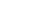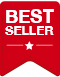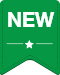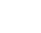×

# Fresh Suki|
Total price: 249 .-|
Total price: 145 .-|
Total price: 52 .-|
Total price: 45 .-|
Total price: 42 .-|
Total price: 24 .-|
Total price: 89 .-|
Total price: 1,055 .-|
Total price: 735 .-|
Total price: 635 .-|
Total price: 635 .-|
Total price: 425 .-|
Total price: 299 .-|
Total price: 299 .-|
Total price: 222 .-|
Total price: 199 .-|
Total price: 185 .-|
Total price: 289 .-|
Total price: 179 .-|
Total price: 102 .-|
Total price: 102 .-|
Total price: 97 .-|
Total price: 89 .-|
Total price: 84 .-|
Total price: 80 .-|
Total price: 77 .-|
Total price: 60 .-|
Total price: 59 .-|
Total price: 59 .-|
Total price: 68 .-|
Total price: 59 .-|
Total price: 49 .-|
Total price: 75 .-|
Total price: 55 .-|
Total price: 55 .-|
Total price: 60 .-|
Total price: 55 .-|
Total price: 49 .-|
Total price: 90 .-|
Total price: 83 .-|
Total price: 82 .-|
Total price: 80 .-|
Total price: 80 .-|
Total price: 80 .-|
Total price: 68 .-|
Total price: 64 .-|
Total price: 59 .-|
Total price: 57 .-|
Total price: 55 .-|
Total price: 55 .-|
Total price: 89 .-|
Total price: 95 .-|
Total price: 52 .-|
Total price: 45 .-|
Total price: 24 .-|
Total price: 49 .-|
Total price: 49 .-|
Total price: 17 .-|
Total price: 45 .-|
Total price: 34 .-|
Total price: 34 .-|
Total price: 34 .-|
Total price: 30 .-|
Total price: 28 .-|
Total price: 28 .-|
Total price: 26 .-|
Total price: 25 .-|
Total price: 22 .-|
Total price: 22 .-|
Total price: 249 .-|
Total price: 145 .-|
Total price: 52 .-|
Total price: 47 .-|
Total price: 45 .-|
Total price: 45 .-|
Total price: 36 .-|
Total price: 34 .-|
Total price: 13 .-

This website uses cookies to improve your experience. MK Restaurant Group Public Company Limited and its subsidiaries would like to get your consent to collect, use, and/or disclose your collected data during our site visit including your personal data. More information
เอ็มเค Live chat
Firstname - Lastname
Mobile Number
EmailStart Chat